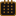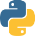# Articles | A collection of Articles, tutorials and thought processes | Olsen Portfolio

Sunday May 22nd, 2022Lambda Functions

What is a lambda function?

In a nutshell, it is an anonymous, inline "throw away" function that can pass any number of arguments (or no arguments at all) but only returns a single executed expression. Further more, it cannot include statements like return, pass, assert, or raise as these will invoke an exception error!

Let's look at a few examples for better clarity! First, we'll start with a simple example that uses a traditional function:

Example 1a (using a traditional function)``````def add_to_number(x):
return x + 7

Output: 15
``````

Now let's rewrite the above example using a lambda function!

Example 1b (using the lambda function)``````add_to_number = lambda x: x + 7

Output: 15
``````

Here is the anatomy of a lambda function:

Lambda function Syntax:

• lambda Argument(s) : Expression

• Argument(s): Value(s) that are passed into the function.
• Expression: a single operation (expression) performed on said argument(s). It's possible to have no expression whatsoever, but is regarded as useless!

• The keyword lambda always comes first, followed by the colon which always separates the argument(s) from the expression!

As mentioned previously, a lambda function can take any number of arguments:

Example 2 (multiple arguments)``````multiply_some_numbers = lambda a, b, c, d: a * b * c * d
print(multiply_some_numbers(1,3,6,10))

Output: 180
``````

It is also possible to execute a lambda function by simply surrounding it (and likewise, trailing argument(s)) using a pair of parentheses like so:

Example 3 (parentheses)``````added_numbers = (lambda x, y: x + y)(2,4)

Output: 6
``````

It is perfectly acceptable to nest lambda functions within other functions!

Example 4 (lambda function nested within a function)``````def myfunc(b):
return lambda a : a * b

result = myfunc(10)
print(result(4))

Output: 40
``````

Lambda function expressions can also be other functions!

Example 5 (a function as an expression)``````(lambda *args : sum(args))(3,5,7) #if printed, the output would be 15
``````

Additionally, you can use a conditional as an expression!

Example 6 (conditional as expression)``````myvar = lambda a,b: a if a > b else b
print(myvar(12,32))

Output: 32
``````

When Should I use a lambda function?

• Small trivial, non complicated tasks requiring a single expression returned.
• Repetitive tasks that are temporary in nature.
• In conjunction with the points above, you can make use of a lambda function when you need to capitalize on Python Closures! Here is an additional closure source as well as this one.

Conclusion

As you can see, while lambda functions are limited, they definitely have their place and can prove quite useful whenever you need a simple, single returned expression in one easy discardable function! ∎